# MSP430 Programming Tutorial Pt/2

In this second part of the MSP430 programming tutorial examples of GPIO register settings will be shown and explained.  Additionally register examples for some of the internal peripherals will be demonstrated and explained.  The first part of the tutorial can be found here.

## Changing the GPIO Registers to your desired configuration

The following examples should help to illustrate how you change the individual bits, as well as multiple bits in the GPIO registers to achieve exactly what you want.  There is a reference to BIT3 and BIT7 being defined as hexadecimal values, these values can be found in the msp430g2253.h header file inside Code Composer Studio, or the MSP430 software provided by Texas Instruments.So by using this statement we only change BIT7 of port 1 to a logic 1 or GPIO P1.7. This is very powerful as it allows individual pins to be configured, without effecting other pins on the same port.  But what if you want to adjust multiple pins to outputs, well that can be achieved quite easily, two ways are illustrated below.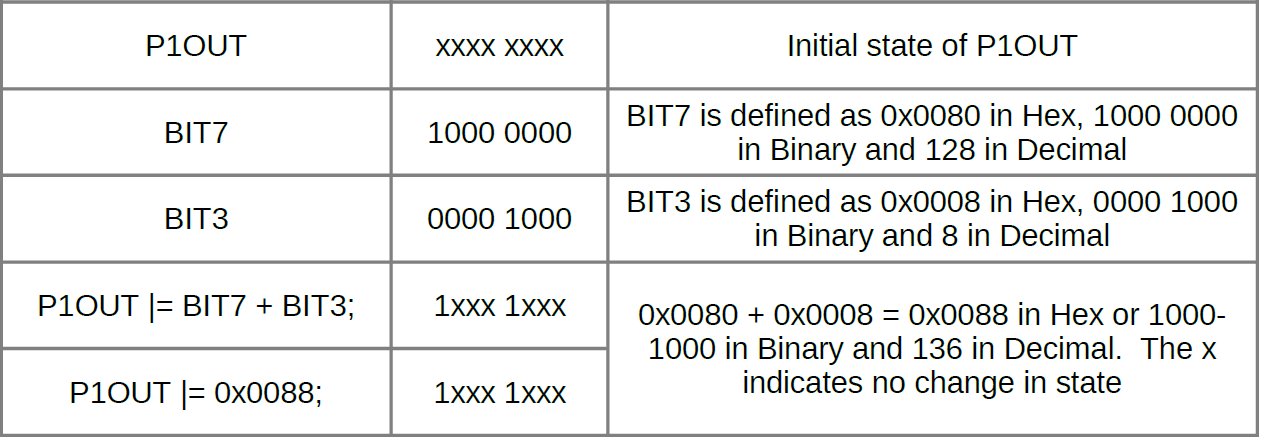So turning a single registry bit or multiple bits to a logic 1 are covered, how about assigning a logic 0 to a single register bit or multiple register bits.  The following images will demonstrate how this is achieved.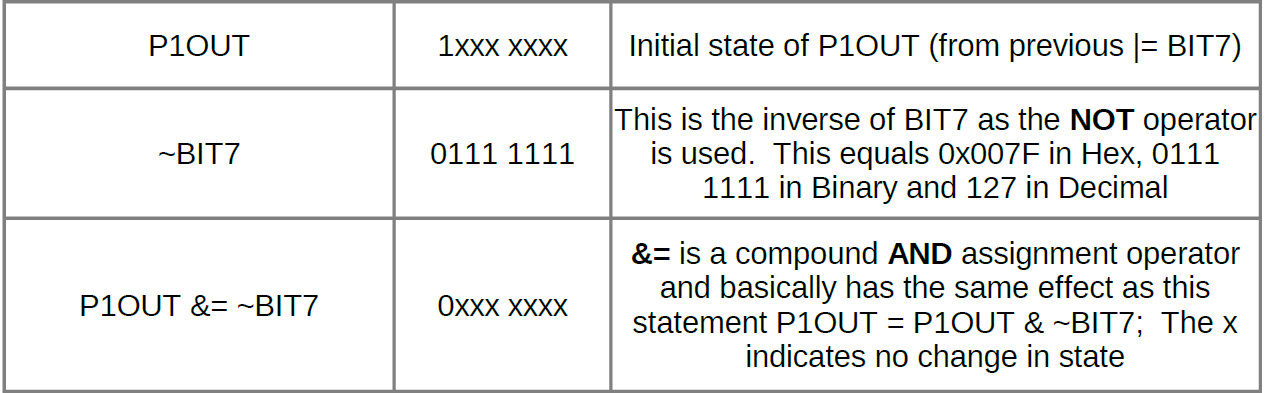And for multiple bits.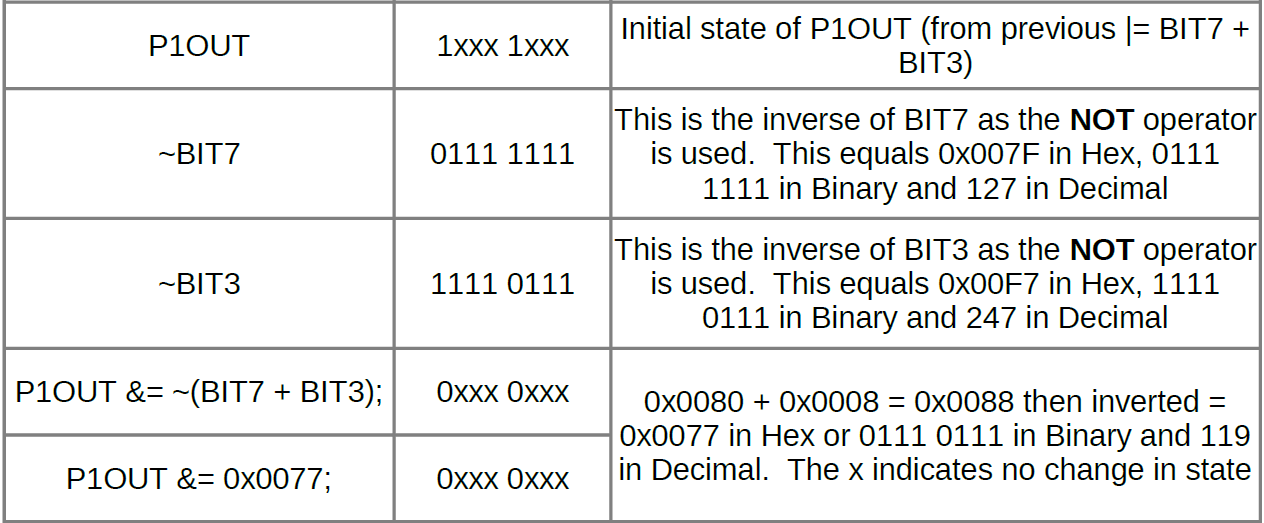There is one more operator that is commonly used on GPIO pin register bits, that is the ^ or XOR bitwise operator.  This is used in many examples on the internet to toggle the LED’s on the launchpad, the example below demonstrates it’s use.Although all these examples are only used with the P1OUT register, the same principles can be applied to all the GPIO port registers.  The examples shown where multiple registers are written to, using a combined hex value will also optimise any code, saving execution time by removing additional arithmetic in the form of an addition.

## Understanding and Changing Peripheral Registers

Understanding how to change bits inside the peripheral registers, is not a great leap in understanding from the GPIO ports.  A few examples will be shown which are based on the ADC peripheral.  The ADC10 Control Register 1 (ADC10CTL1) will be used as the example register, but all registers will follow the same principle.  The image below is extracted from the MSP430 family guide and shows the ADC10CTL1 register.So what we can see here is the register is a 16 bit register and the bits are split into blocks which correspond to different parameters.  I have covered what these individual blocks do in a previous tutorial dedicated to the MSP430 ADC, found here.  It can be seen that the blocks correspond to certain bits inside the register, for example INCHx occupies Bits 12-15 of the register and ADC10DIVx occupies Bits 5-7 of the register.  To illustrate this we could view the 16 bit register in binary form:

 INCHx occupies the bits shown in bold 0000 0000 0000 0000 ADC10DIVx occupies the bits shown in bold 0000 0000 0000 0000

As with the GPIO port pins Texas Instruments have provided defines in the header files, so it is not necessary to remember the binary or hexadecimal values for the register settings. The names used for these register settings are shown above i.e. INCHx and ADC10DIVx.  As INCHx occupies four Bits it therefore has 16 possible combinations and ADC10DIVx has 8, hence the small x after each name.

The next two images are again extracted from the MSP430 family guide and show how the bit combinations correspond to different parameters.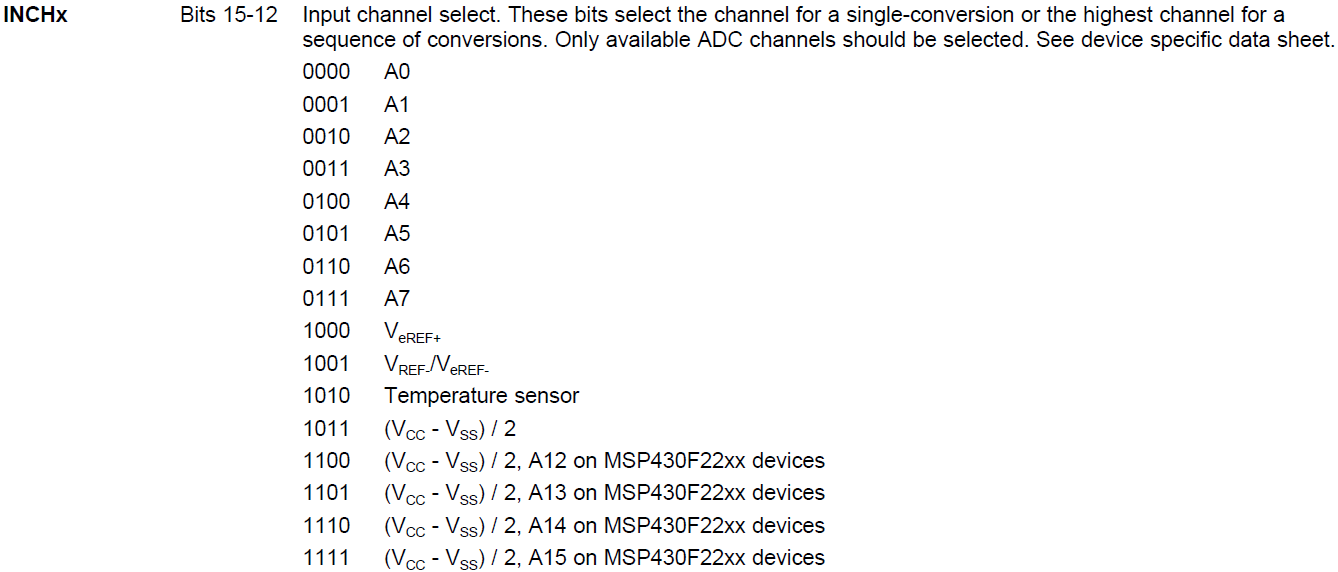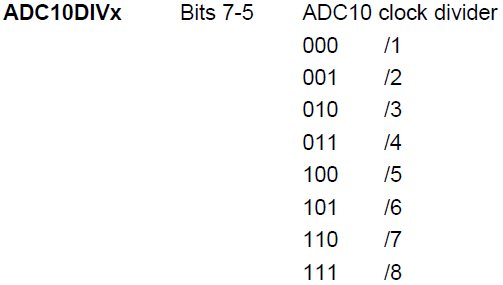So now lets look at a command to set the ADC10CTL1 register using the parameters INCHx and ADC10DIVx.

The code snippet above basically adds the two parameters together and assigns them to the register using a compound OR assignment operator.  Now lets look at this in binary form, which will help shed some light on the process.So by using this command the register parameters can be set individually, or multiple parameters can be set in one go.  As with the GPIO port settings a hexadecimal value can be used to set the parameters as well.

The code snippet above achieves the same result as well as being more efficient, however the code becomes much more obfuscated.

That covers setting the register bits to 1, how about setting the register bits to 0.  This is achieved in the same way as with the GPIO, the image below illustrates the operation.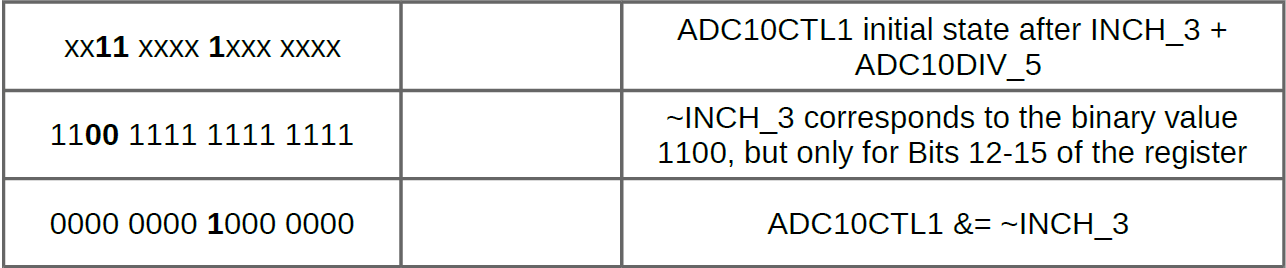I take great care when writing all the tutorials and articles, ensuring all the code is fully tested to avoid issues for my readers.  All this takes time and a great deal of work, so please support the site by using the Adfly links etc.  If you have found this useful or have any problems implementing, please feel free to leave a comment and I will do my best to help.

# MSP430 Programming Tutorial Pt/1

In this MSP430 programming tutorial part 1 some of basic C operators used for programming the MSP430 will be looked at.  The GPIO port registers will then be looked at in greater detail.  In part 2 example code for the GPIO registers will be shown and explained, as well as examples for the the ADC peripheral register.   The MSP430G2253 Launchpad will be used as the reference microcontroller, the primary IDE used is Code Composer Studio (CCS). The MSP430G series family guide, as well as other useful information can be downloaded directly from Texas Instruments webpage here.

## C Language Bitwise Operators

If you are familiar with Bitwise operators, skip this section and and start with the MSP430 GPIO ports section further down this page.

Some basic C language bitwise operators will be looked at first, then how these apply to GPIO ports will be demonstrated.  The C language has 8 types of operators and bitwise operators are 1 of these.  The bitwise operators are fairly easy to understand and if you have ever looked at logic gates and truth tables, then some of these will be immediately recognisable. For the following examples two variables will be used a and b, they will also be assigned values; Decimal: = 48 and b = 24, Binary: a = 0011 0000 and b = 0001 1000.

#### Bitwise Operator &

 a 0011 0000 b 0001 1000 a&b 0001 0000

The binary AND operator copies a bit or logic 1 to the result, only if a logic 1 exits in both operands.  So the result of a&b = 0001 0000 or 16 in decimal.

#### Bitwise Operator |

 a 0011 0000 b 0001 1000 a|b 0011 1000

The binary OR operator copies a bit or logic 1 to the result, if it exists in either operand.  So the result of a|b = 0011 1000 or 56 in decimal.

#### Bitwise Operator ^

 a 0011 0000 b 0001 1000 a^b 0010 1000

The binary XOR operator copies a bit or logic 1 to the result, if it exists in one operand as a logic 1, but not both.  So the result of a^b = 0010 1000 or 40 in decimal.

#### Bitwise Operator ~

 a 0011 0000 ~a 1100 1111

The binary NOT operator effectively flips the bits, so 1’s become 0s and vice versa.  So the result of ~a = 1100 1111 or -48 in decimal (signed variable) or 207 in decimal (unsigned variable).

#### Bitwise Operator <<

 a 0011 0000 a = a<<2 1100 0000

The binary LEFT SHIFT operator moves the operands bits left, by the number of bits specified, which is this case is 2.  So the result of aa<<2 = 1100 0000 or 192 in decimal.  This also effectively multiplies the value of a by a factor of 4.

#### Bitwise Operator >>

 a 0011 0000 a = a>>2 0000 1100

The binary RIGHT SHIFT operator moves the operands bits right, by the number of bits specified, which is this case is 2.  So the result of a = a>>2 = 0000 1100 or 12 in decimal. This also effectively divides the value of a by a factor of 4.

## MSP430 GPIO Ports

Looking at the 20 pin MSP430G2253 supplied with the Launchpad, it has two GPIO ports. Both ports have 8 GPIO pins numbered as follows, port 1 pins P1.0 to P1.7 and port 2 pins P2.0 to P2.7.  The image below is extracted from the MSP430G2253 datasheet.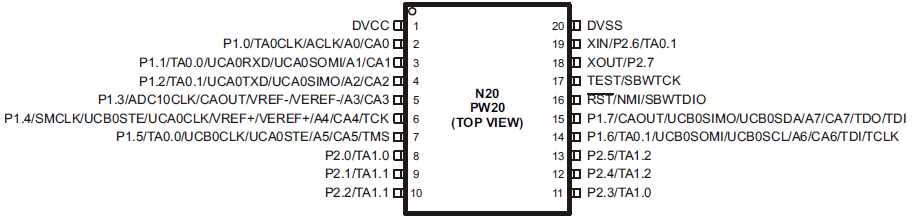All the individual GPIO pins can be configured to connect to internal peripherals, for example, providing a connection for the ADC to an external source, or providing the output from Timer module in the shape of a PWM signal.  The GPIO’s as the acronym tells also provide General Purpose Input and Output operations.  Not all of the GPIO pins can be configured to be used by all the internal peripherals, a detailed list of how the pins can configured can be found in the datasheet for that particular microcontroller.  The image below again shows an extract from the MSP430G2253 datasheet, illustrating the GPIO pins P1.0 and P1.1 and their individual associated peripheral functions.Defining how each of the eight GPIO pins are configured for each port, is achieved by individual registers.

#### PxIN Input Register

The PxIN register reflects the value of the signal being input into the GPIO pin, when configured as an I/O function.  So by reading this value you can determine if there is a logic 0 or a logic 1 on the input.  Bit = 0: The input is low, Bit = 1: The input is high.  A statement using this function for GPIO port 2 and pin P2.4, could look like this if ((P2IN & Bit4) == BIT4);.

#### PxOUT Output Register

The PxOUT register determines the value output to the GPIO pin, when the pin is configured as an I/O function.  The PxOUT register works in conjunction with the PxREN as follows:

Pullup/pulldown resistor disabled: Bit = 0: The output is low, Bit = 1: The output is high.

Pullup/pulldown resistor enabled: Bit = 0: The pin is pulled down, Bit = 1: The pin is pulled up.

A statement using this function for GPIO port 1 and pin P1.4, could look like this P1OUT &= ~BIT4.

#### PxREN Pullup/Pulldown Resistor Register

The PxREN register enables or disables the internal pullup/pulldown resistor, which corresponds to the individual I/O pin.  Bit = 0: Pullup/pulldown resistor disabled, Bit = 1: Pullup/pulldown resistor enabled.  A statement using this function for GPIO port 1 and pin P1.5, could look like this P1REN |= BIT5.

#### PxDIR Direction Register

The PxDIR register selects the direction of the I/O pin, whether it will be an input or an output.  This is regardless of the selected function of the pin.  Bit = 0: The port pin is switched to input direction, Bit = 1: The port pin is switched to output direction.    A statement using this function for GPIO port 1 and pin P1.3, could look like this P1DIR |= BIT3.

#### PxSEL and PxSEL2 Function Select Registers

The PxSEL and PxSEL2 registers allow the individual GPIO pins to be associated with the internal peripheral module functions, or simply left as standard I/O ports.  The image below was extracted from the MSP430 family guide.A statement using this function for GPIO port 2 and pin P2.1, could look like this P2SEL |= BIT1;.  When using these registers, it is important to consult the datasheet and pin schematics, for the specific device.

#### P1IFG, P2IFG Interrupt Flag Registers

Only GPIO ports 1 and 2 have interrupt functionality.  The P1IFG and P2IFG registers hold the interrupt flag for the corresponding I/O pin, the interrupt flag is set when the selected input signal edge occurs at the pin.  Bit = 0: No interrupt is pending, Bit = 1: An interrupt is pending.  A statement using this function for GPIO port 1 and pin P1.1, could look like this P1IFG &= ~BIT1;.

#### P1IES, P2IES Interrupt Edge Select Registers

The P1IES and P2IES registers allow the interrupt edge type to be selected for each I/O pin. Bit = 0: The PxIFGx flag is set with a low to high transition, Bit = 1: The PxIFGx flag is set with a high to low transition.  A statement using this function for GPIO port 1 and pin P1.1, could look like this P1IES &= ~BIT1;.

#### P1IE, P2IE Interrupt Enable Registers

The P1IE and P2IE register bit enables the associated PxIFG interrupt flag.  Bit = 0: The interrupt flag is disabled, Bit = 1: The interrupt flag is enabled.  A statement using this function for GPIO port 1 and pin P1.1, could look like this P1IE |= BIT1;.

Texas Instruments also recommends configuring unused pins as I/O function, output direction, and left unconnected to prevent a floating input and reduce power consumption.

So how to change the GPIO Registers to what you want, well part 2 of this tutorial will make the GPIO settings clear and hopefully easy.  Additionally an ADC register will be explained and demonstrated.

I take great care when writing all the tutorials and articles, ensuring all the code is fully tested to avoid issues for my readers.  All this takes time and a great deal of work, so please support the site by using the Adfly links etc.  If you have found this useful or have any problems implementing, please feel free to leave a comment and I will do my best to help.